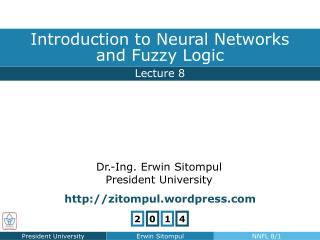DownloadDownload PresentationQuestion: Is it warm in here?

# Question: Is it warm in here?

Télécharger la présentation## Question: Is it warm in here?

- - - - - - - - - - - - - - - - - - - - - - - - - - - E N D - - - - - - - - - - - - - - - - - - - - - - - - - - -
##### Presentation Transcript

1. yes fairly warm maybe a little no not really Fuzzy Logic Implication and Inference Question: Is it warm in here? • Answer • IF room is warmTHEN set cooling power to 500 watts

2. Fuzzy Logic Implication and Inference Fuzzy Inference Premise Consequence • IF room is warm,THEN set cooling power to 500 watts. Measurement • The room temperature is 21 °C. • Set cooling at 280 watts. Action

3. Fuzzy Logic Fuzzy Logic Terminologies • Fuzzy Universe: range of all possible values to a chosen variable • Fuzzy Set: set with fuzzy boundaries • Fuzzy Membership Function: used to define fuzzy set • Fuzzy Set Operations: not, or, and • Fuzzy Logic Operators: the realization of set operation • Fuzzy Variable or Fuzzy Linguistic Value: variable assigned to fuzzy sets, such as: tall, high, fast • Fuzzy Linguistic Variable: variable that takes the value of certain fuzzy variable, such as: person, pressure, velocity • Fuzzy Rules: conditional statements that relates fuzzy sets

4. Fuzzy Logic Membership Function Fuzzy Membership Function • Single-Valued (Singleton) • Trapezoidal • Triangular • Sigmoid • Gaussian • etc., as can be seen later in Fuzzy Toolbox

5. Fuzzy Logic Membership Function Fuzzy Membership Function: Tall People Degree ofMembership FuzzyMembershipFunction Fuzzy Universe

6. Fuzzy Logic Membership Function Fuzzy Membership Function: Around Noon Trapezoid Triangular Smooth trapezoid Smooth triangular

7. Fuzzy Logic Membership Function A B Fuzzy Set Operations Min-Max Operators OR AND NOT

8. Fuzzy Logic Membership Function Fuzzy Logic Operators s-Norm: is monotonous associative operator with t-Norm: is monotonous associative operator with FL-OR must resemble s-Norm FL-AND must resemble t-Norm Monotony Associativity

9. Fuzzy Logic Membership Function Fuzzy Logic Operators NOT Operator OR and AND Operators Max Min Algebraic sum Algebraic product Bounded sum Bounded product

10. Fuzzy Logic Membership Function Fuzzy Logic Operators • Relation between s-Norm and t-Norm: de Morgan’s Law Min-Max Algebraic ........? (Prove) Bounded........? (Prove)

11. Fuzzy Logic Membership Function Homework 7 • The following membership functions are given:“Temperature is low” μTl(T),“Temperature is middle” μTm(T). • For all three possible realizations of FL-Operators (Min-Max, Algebraic, Bounded), draw the membership functions of:(i) “Temperature is low” AND “Temperature ismiddle”;(ii) “Temperature is low” OR “Temperature is middle”.

12. Fuzzy Logic Membership Function Homework 7A • The following membership functions are given:“Pressure is moderate” μPm(P),“Pressure is high” μPh(P). • For all three possible realizations of FL-Operators (Min-Max, Algebraic, Bounded), draw the membership functions of:(i) “Pressure is moderate” AND “Pressure is not high”;(ii) “Pressure is not moderate” OR “Pressure is high”.